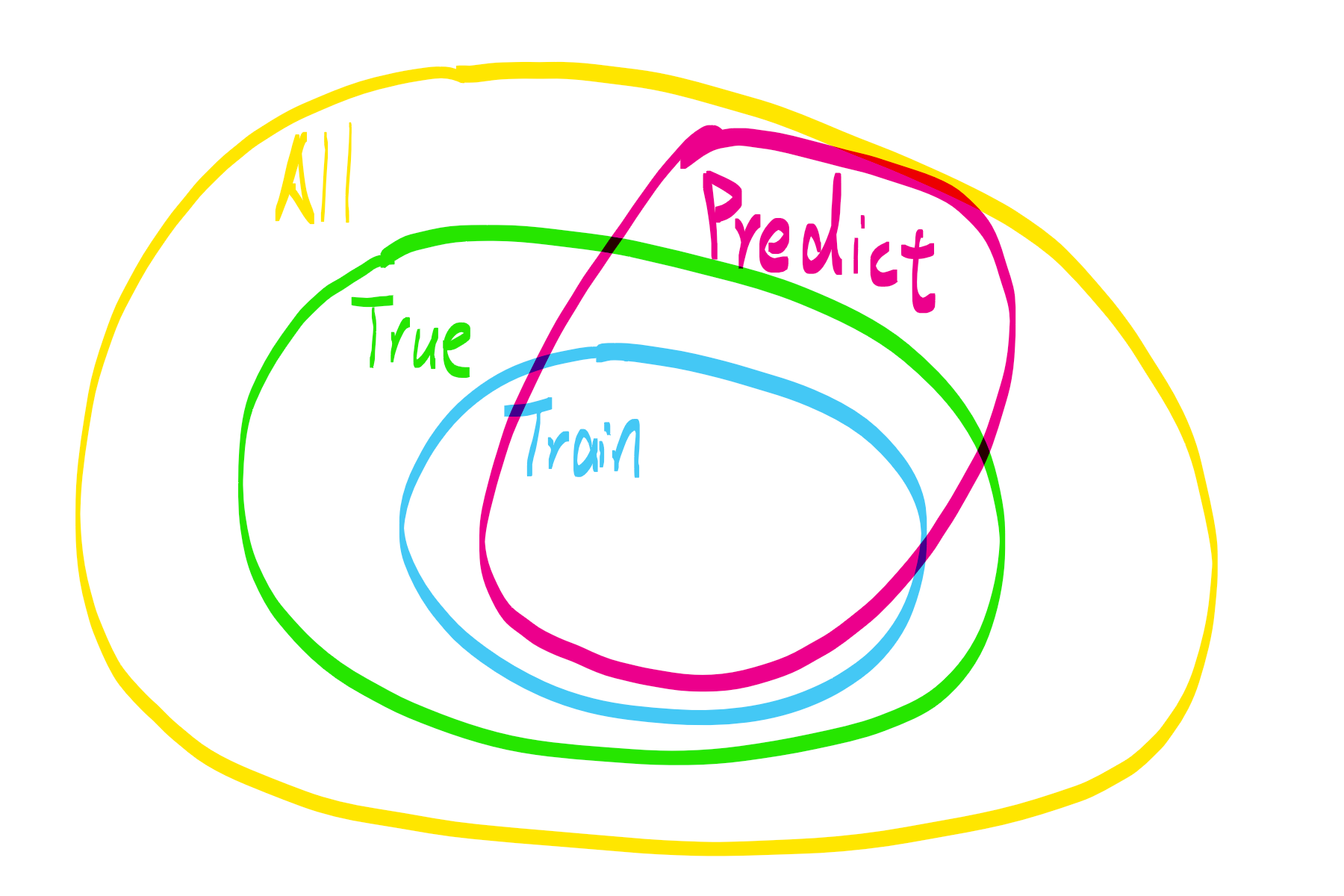# ML Course Notes 02

## Lecture 06: Classification and Representation

### Classification

Note: This part is focusing on the binary classification problem

• The classification problem is just like the regression problem, except that the values we now want to predict take on only a small number of discrete values. In short, the target value is discrete.
• To attempt classification, one method is to use linear regression(here we are talking about regression methods without penalty) and map all predictions. But this won’t work very well, some of the reasons are as follows:
• Decision boundary is hard to choose
• It’s very sensitive to outliers

### Hypothesis Representation

By ignoring the fact that y is discrete-valued, it’s able to use our old linear regression algorithm to try to solve the (binary) classification problem.

What we need is Sigmoid Function(also called Logistic Function) to map the results.

$h_\theta (x)$ actually give us the probability that our output is 1.

### Decision Boundary

The decision boundary is the line that separates the area where y = 0 and where y = 1. It is created by our hypothesis function.

Note that the input to the sigmoid function g(z) doesn’t need to be linear, and could be a function that describes a circle or any shape to fit our data. I guess it’s like a hyperplane. For binary classification problem, the hyperplane is in 1d which result in a line or a curve.

### cost function

The same cost function that used for linear regression won’t work because the Logistic Function will cause the output to be wavy, causing many local optima. In other words, it will not be a convex function.

Cost function for logistic regression looks like: %

For positive samples, cost equals 0 when our hypothesis output 1, the cost will approach infinity if hypothesis approaches 1.

Note that writing the cost function in this way guarantees that J(θ) is convex for logistic regression.

### Simplified Cost Function and Gradient Descent

We can compress our cost function’s two conditional cases into one case:

• when $y=1$ (Positive sample), $(1 - y) \log(1 - h_\theta(x))$ will be 0 and won’t effect the result.
• When $y=0$ (Negative sample), $- y \; \log(h_\theta(x))$will be 0 and won’t effect the result.

Vectorized implement Cost function is: %

Based on that simplified Cost Function, gradient descent algorithm can also be simplified to:

Some much advanced optimization are provided. Such as “SGD”,”Conjugate gradient”, “BFGS”, and “L-BFGS”. Ng suggest that you should not write these more sophisticated algorithms yourself (unless you are an expert in numerical computing) but use the libraries instead.

SO just use them, be a api_caller is fine.

### Multiclass Classification

In a word: One-vs-all. pass;

## Lecture 07: Solving the Problem of Overfitting

Hereby is a venn diagram I draw when I am reading something about NFL.Look at the parts where Predict-True(the sub is aggregate subtraction), it is actually overfitting parts. Overfitting can not be solved, because the train set is not independent, identical distributed(i.i.d) with true set.(Train-Predict is underfitting part)

But it’s possible to reduce overfitting. There are two main options to address the issue of overfitting:

1. Reduce the number of features
• Manually select which features to keep.
• Use a model selection algorithm.
2. Regularization
• Keep all the features, but reduce the magnitude of parameters $\theta_j$.
• Regularization works well when we have a lot of slightly useful features.

### Modify the Cost Function

The aim is to reduce the weight that some of the terms in our function, which may able to address the issue of overfitting.

We can regularize all of our theta parameters in a single summation as:

$\lambda$ is the regularization parameter. It determines how much the costs of our theta parameters are inflated.

Actually this is L2 regulartion, and this linear regression method is called Ridge. While LASSO use L1 regulartion. Lasso shrinks the less important feature’s coefficient to zero thus, removing some feature altogether.

Using the above cost function with the extra summation, we can smooth the output of our hypothesis function to reduce overfitting. If lambda is chosen to be too large, it may smooth out the function too much and cause underfitting.

Regularization makes

• “simpler” hypothesis
• less prone to overfitting
• (smaller and better)

### Regularized Linear Regression

With the modified cost function above, we can also get a modified gradient desent algorithm:

Modified normal equation algorithm is also easy to figuare out

### Regularized Logistic Regression

We can regularize logistic regression in a similar way that we regularize linear regression.

pass;

removed;

## Lecture 08: Neural Networks:Representation

To address non-linear hypothese issures, we will represent a hypothesis function using neural networks.

Neural network:

• Origins: Algorithms that try to mimic the brain.
• At a very simple level, neurons are basically computational units that take inputs (dendrites) as electrical inputs (called “spikes”) that are channeled to outputs (axons).
• I once wrote a detailed blog about neural networks in chinese which can be visited by click here

I am quite familar with basic NN, so… pass;

### Programming Assignment: Multi-class Classification and Neural Networks

removed;